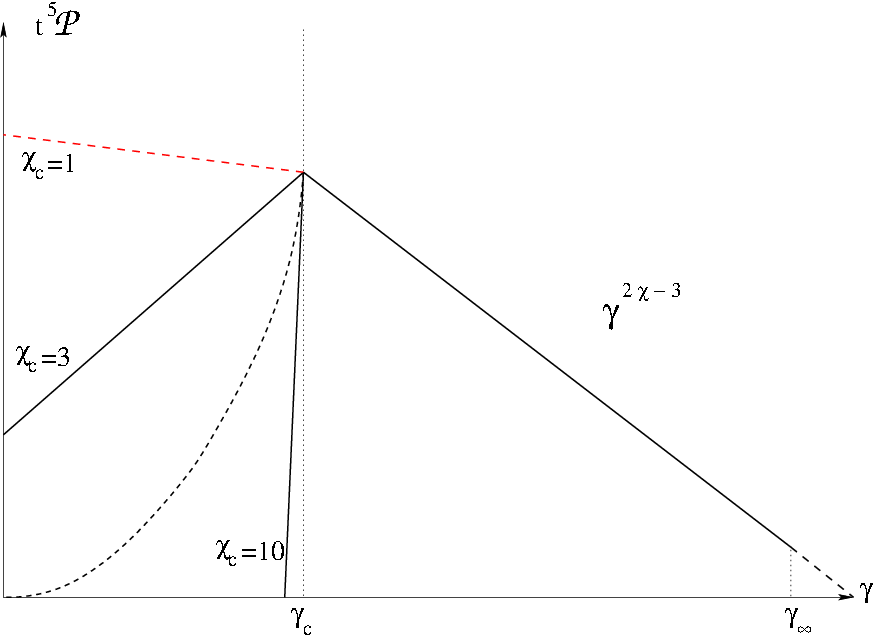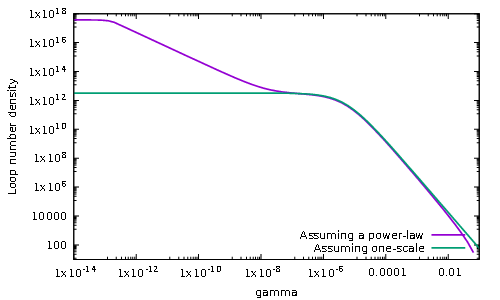# The shape of cosmic string loop production functions

We have recently made public a rather technical paper in which we explicitly and unambiguously calculate the cosmic string loop number density distribution in our universe coming from various motivated loop production functions. Such a density distribution crucially determines the spectrum of their emitted gravitational waves, a smooking gun for their potential discovery.

Together with Pierre Auclair, Mairi Sakellariadou and Danièle Steer, we have carefuly explored in Ref. the consequences of changing the slope of the Polchinski-Rocha loop production function to the actual observable cosmic string loop distribution. This is the parameter $$\chi$$ in the next plot:For the cases we have referred to as “sub-critical”, $$\chi < (3 \nu - 1)/2$$, corresponding to steep slopes in the previous figure, we recover the results of Ref. . The parameter $$\nu$$ encodes the growing rate of the scale factor $$a(t)$$, namely, we assume $$a(t) \propto t^\nu$$.

For shallower slopes, the so-called “critical” and “super-critical” cases, $$\chi \ge (3\nu-1)/2$$, we find that either the loop distribution incessantly grows, or, with some regularisation, reaches a stationnary distribution whose shape depends on what happens on the larger length scales. This is best illustrated by the following plot:It compares the loop number density produced by assuming an infinitely sharp loop production function peaked at $$\gamma = 0.1$$ (green curve) with a (regularised) super-critical Polchinski-Rocha distribution having $$\chi > 0.25$$ (purple curve), in the radiation era ($$\nu=1/2$$). As this plot shows, the gravitational backreaction scale, $$\gamma_\mathrm{c}$$, at which the loop production function is cut always matters and is responsible for the plateau on the purple curve at small $$\gamma$$ values. The existence of a second plateau around $$\gamma \simeq 10^{-6}$$ comes from the Infra-Red sensitivity of all super-critical loop production functions.

### References

•  Auclair P, Ringeval C, Sakellariadou M and Steer D 2019 Cosmic string loop production functions JCAP 1906 015
Abstract: arXiv:1903.06685 10.1088/1475-7516/2019/06/015

•  Lorenz L, Ringeval C and Sakellariadou M 2010 Cosmic string loop distribution on all length scales and at any redshift JCAP 1010 003
Abstract: arXiv:1006.0931 10.1088/1475-7516/2010/10/003